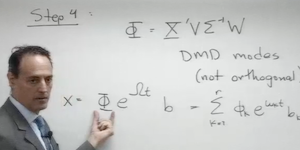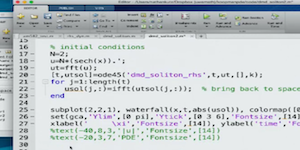# Dynamic Mode Decomposition

## Introduction to DMD theory

### Lecture 1### [ Part 1 ] [ Part 2 ]

Dynamic Mode Decomposition: This lecture provides an introduction to the Dynamic Mode Decomposition (DMD). The focus is on approximating a nonlinear dynamical system with a linear system.

MATLAB CODE

### Lecture 2### [ view ]

Koopman Theory: This lecture generalizes the DMD method to a function of the state-space, thus potentially providing a coordinate system that is intrinsically linear.

MATLAB CODE